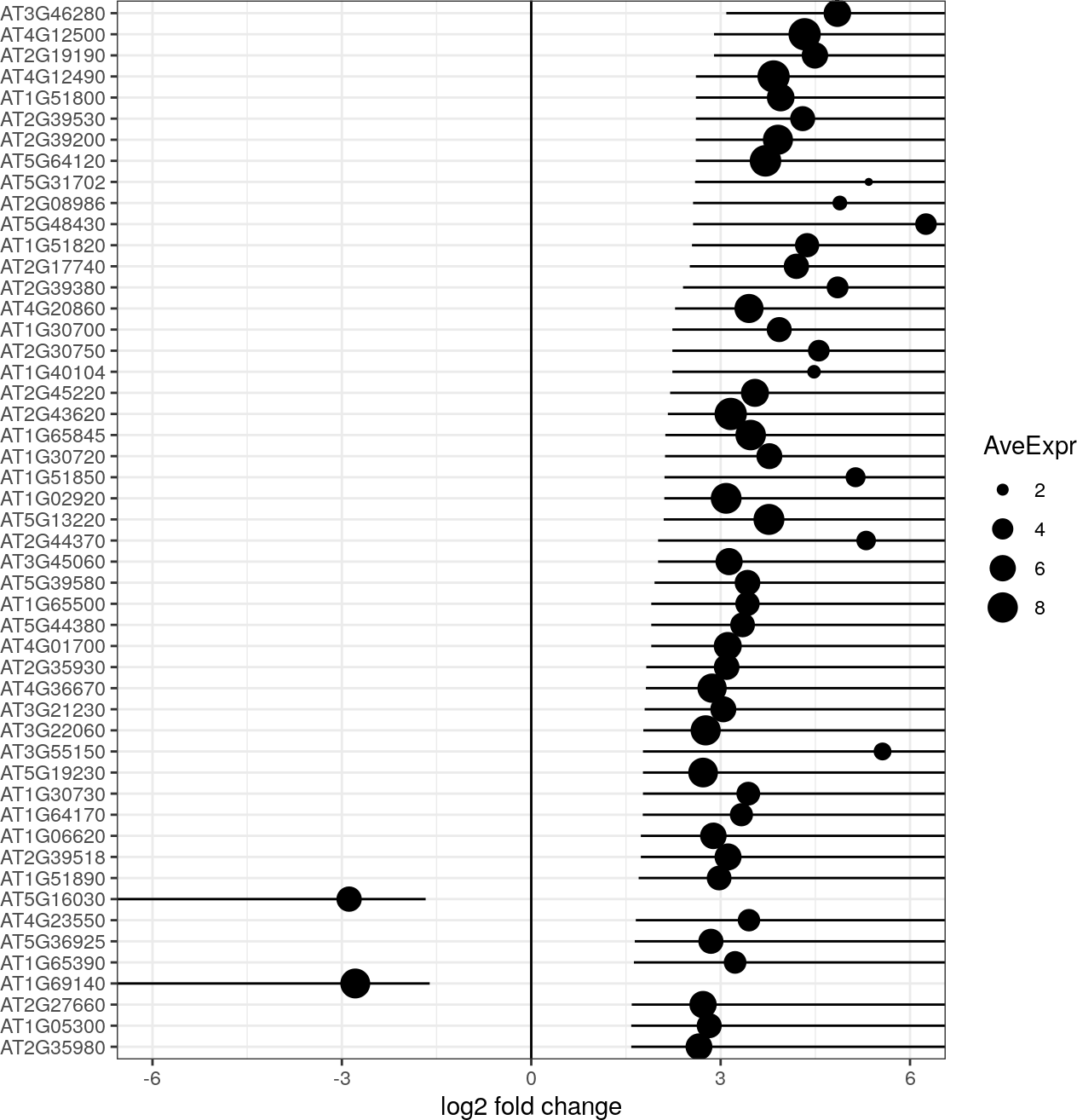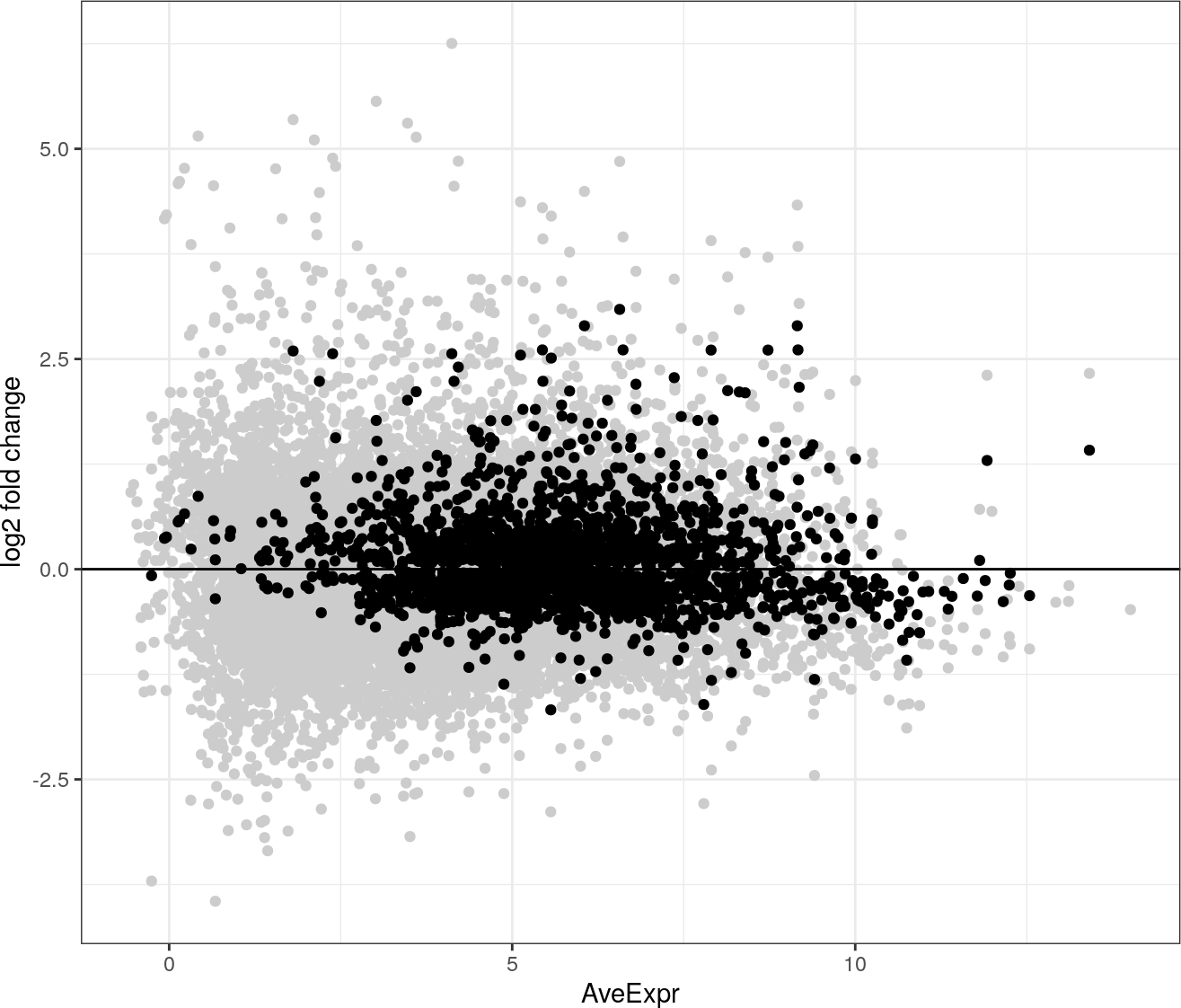# Contents

This document shows typical Topconfects usage with limma, edgeR, or DESeq2.

The first step is to load a dataset. Here, we’re looking at RNA-seq data that investigates the response of Arabodopsis thaliana to a bacterial pathogen. Besides the experimental and control conditions, there is also a batch effect. This dataset is also examined in section 4.2 of the `edgeR` user manual, and I’ve followed the initial filtering steps in the `edgeR` manual.

``````library(topconfects)

library(NBPSeq)
library(edgeR)
library(limma)

library(dplyr)
library(ggplot2)

data(arab)

# Retrieve symbol for each gene
info <-
AnnotationDbi::select(
org.At.tair.db::org.At.tair.db,
rownames(arab), "SYMBOL") %>%
group_by(TAIR) %>%
summarize(
SYMBOL=paste(unique(na.omit(SYMBOL)),collapse="/"))
arab_info <-
info[match(rownames(arab),info\$TAIR),] %>%
select(-TAIR) %>%
as.data.frame
rownames(arab_info) <- rownames(arab)

# Extract experimental design from sample names
Treat <- factor(substring(colnames(arab),1,4)) %>% relevel(ref="mock")
Time <- factor(substring(colnames(arab),5,5))

y <- DGEList(arab, genes=as.data.frame(arab_info))

# Keep genes with at least 3 samples having an RPM of more than 2
keep <- rowSums(cpm(y)>2) >= 3
y <- y[keep,,keep.lib.sizes=FALSE]
y <- calcNormFactors(y)``````

# 1 limma analysis

## 1.1 Standard limma analysis steps

``````design <- model.matrix(~Time+Treat)
design[,]``````
``````##   (Intercept) Time2 Time3 Treathrcc
## 1           1     0     0         0
## 2           1     1     0         0
## 3           1     0     1         0
## 4           1     0     0         1
## 5           1     1     0         1
## 6           1     0     1         1``````
``````fit <-
voom(y, design) %>%
lmFit(design)``````

## 1.2 Apply topconfects

Find largest fold changes that we can be confident of at FDR 0.05.

``````confects <- limma_confects(fit, coef="Treathrcc", fdr=0.05)

confects``````
``````## \$table
##    confect effect AveExpr name      SYMBOL
## 1  3.090   4.849  6.567   AT3G46280
## 2  2.895   4.331  9.155   AT4G12500
## 3  2.895   4.493  6.053   AT2G19190 FRK1/SIRK
## 4  2.608   3.839  9.166   AT4G12490 AZI3
## 5  2.608   3.952  6.615   AT1G51800 IOS1
## 6  2.608   4.299  5.440   AT2G39530 CASPL4D1
## 7  2.606   3.908  7.899   AT2G39200 ATMLO12/MLO12
## 8  2.606   3.710  8.729   AT5G64120 AtPRX71/PRX71
## 9  2.595   5.346  1.807   AT5G31702
## 10 2.563   4.888  2.383   AT2G08986
## ...
## 2184 of 16526 non-zero log2 fold change at FDR 0.05
## Prior df 18.2``````

## 1.3 Looking at the result

Here the usual `logFC` values estimated by `limma` are shown as dots, with lines to the `confect` value.

``confects_plot(confects)```confects_plot_me` overlays the confects (black) on a Mean-Difference Plot (grey) (as might be produced by `limma::plotMD`). As we should expect, the very noisy differences with low mean expression are removed if we look at the confects.

``confects_plot_me(confects)``Let’s compare this to the ranking we obtain from `topTable`.

``````fit_eb <- eBayes(fit)
top <- topTable(fit_eb, coef="Treathrcc", n=Inf)

rank_rank_plot(confects\$table\$name, rownames(top), "limma_confects", "topTable")``````